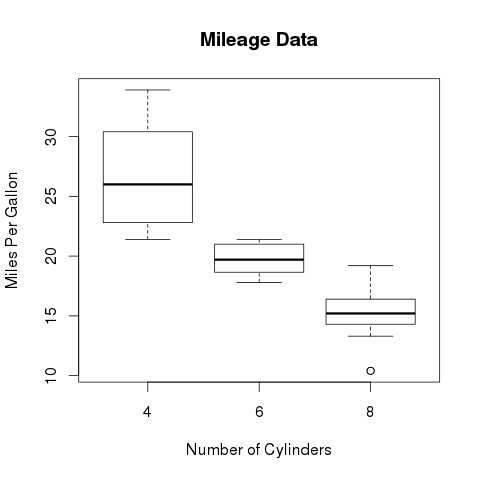# R语言 箱线图

R语言中使用boxplot()函数来创建箱线图。

## 语法

```boxplot(x, data, notch, varwidth, names, main)
```

• x是向量或公式。
• 数据是数据帧。
• notch是逻辑值。 设置为TRUE以绘制凹口。
• varwidth是一个逻辑值。 设置为true以绘制与样本大小成比例的框的宽度。
• names是将打印在每个箱线图下的组标签。
• main用于给图表标题。

## 例

```input <- mtcars[,c('mpg','cyl')]
```

```                   mpg  cyl
Mazda RX4         21.0   6
Mazda RX4 Wag     21.0   6
Datsun 710        22.8   4
Hornet 4 Drive    21.4   6
Valiant           18.1   6
```

## 创建箱线图

```# Give the chart file a name.
png(file = "boxplot.png")

# Plot the chart.
boxplot(mpg ~ cyl, data = mtcars, xlab = "Number of Cylinders",
ylab = "Miles Per Gallon", main = "Mileage Data")

# Save the file.
dev.off()
```## 带槽的箱线图

```# Give the chart file a name.
png(file = "boxplot_with_notch.png")

# Plot the chart.
boxplot(mpg ~ cyl, data = mtcars,
xlab = "Number of Cylinders",
ylab = "Miles Per Gallon",
main = "Mileage Data",
notch = TRUE,
varwidth = TRUE,
col = c("green","yellow","purple"),
names = c("High","Medium","Low")
)
# Save the file.
dev.off()
```## 领取限量阿里云产品优惠

#### 今日限时秒杀 & 新用户专享1折起 & 爆款产品5折起